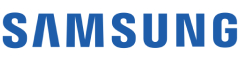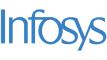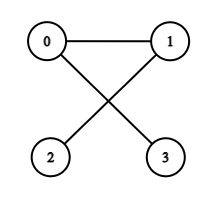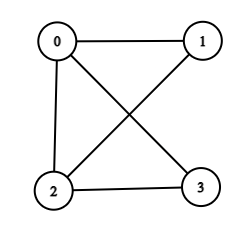New update is available. Click here to update.

# Is Graph Bipartite?

Medium0/801 upvotes+4 more

## Problem Statement

#### You are given an undirected graph consisting of ‘N’ nodes from 0 to ‘N’ - 1. You are given a list ‘EDGES’ of size ‘M’, consisting of all the edges of this undirected graph. Determine whether the given graph is Bipartite or not.

##### Note:
``````The graph has no self-edges, no parallel edges.

The graph may not be connected.

A graph is bipartite if the nodes of the graph can be partitioned into two independent sets A and B such that every edge in the graph connects a node in set A and a node in set B.
``````
##### For Example,
``````If ‘N’ = 4, ‘M’ = 5, edgeList = [ [0, 1],[0, 3],[1, 2] ].
````````````Here, you can see that the graph is bipartite as we can divide the nodes in two sets as follows:
setA = [0, 2].
setB = [1, 3].

In the graph, you can see that every edge in the graph connects a node in set A and a node in set B.
Hence, the output is “Yes”.
``````
Detailed explanation ( Input/output format, Notes, Images )
##### Constraints:
``````1 <= T <= 10
1 <= N <= 500
1 <= M <= (N * (N - 1)) / 2

Time limit: 1 sec
``````
##### Sample Input 1 :
``````2
4 3
0 1
0 3
1 2
4 5
0 1
0 3
1 2
2 3
0 2
``````
##### Sample output 1 :
``````Yes
No
``````
##### Explanation For Sample Output 1:
``````For the first test case, the graph will be:
````````````Here, you can see that the graph is bipartite as we can divide the nodes into two sets as follows:
setA = [0, 2].
setB = [1, 3].

In the graph, you can see that every edge in the graph connects a node in set A and a node in set B.
Hence, the output is “Yes”.

For the second test case, the graph will be:
````````````Here, you cannot divide the nodes into two independent sets A and B such that every edge in the graph connects a node in set A and a node in set B. Hence, the output is “No”.
``````
##### Sample Input 2 :
``````2
4 4
0 1
0 2
0 3
2 3
3 3
0 2
1 0
1 2
``````
##### Sample output 2 :
``````No
No
``````AutocompleteConsole